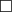# Sets of Functions

Consider the set of all functions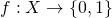. Each subset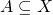has such a function, namely its characteristic function,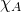; moreover, given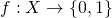we can define the set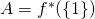and see that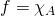. So the functionscorrespond to subsets of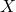.

Definition. Given sets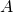,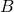, define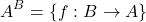to be the set of all functions fromto.

This notation connects with our powerset notation by writing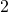for the set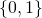(which has two elements).

Theorem. Ifhas exactlyelements andhas exactly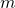elements, then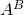has exactly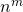elements.

Proof. To define a functionrequires choosing, for each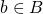, one of theelements of.

Each time we make such a choice, there areoptions; and we have to make the choicetimes. So in total there arepossibilities.